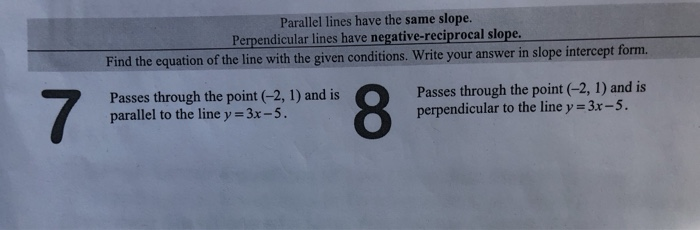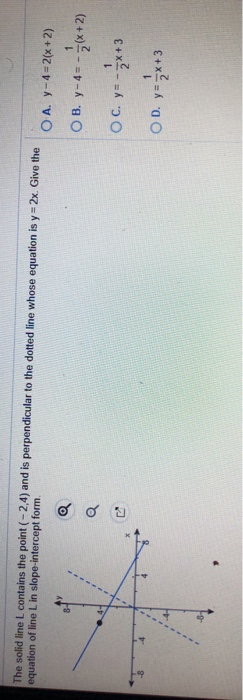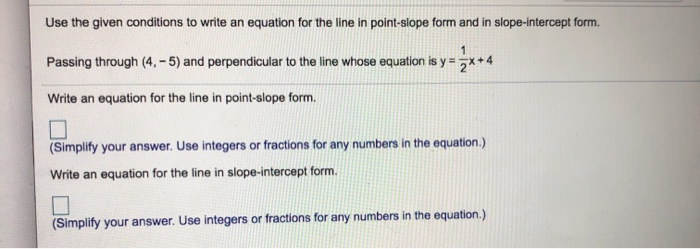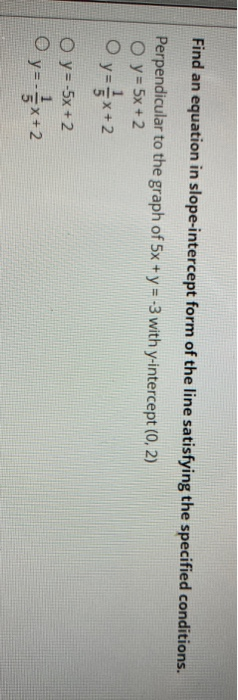# Find the slope of a line perpendicular to the line

Find the slope of a line perpendicular to the line
y=x

Use the slope-intercept form of a linear equation to write the equation of each line with the given slope and y-intercept.

slope -3; y-intercept (0, -1/5)

write the equation of the line passing through the given points. write the equation in standard form Ax+By=C

(8,-3) AND (4,-8)

Write an equation of each line. Write the equation in the form x=a y=b or y =mx+b

Through (-2,-3): perpendicular to 3x+2y =5

Find the equation of each line. Write the equation in standard form unless indicated otherwise.

Through (3,5) perpendicular to the line 2x-y=8

Solve each system by graphing.
-x +3y =6
3x - 9y = 9

These two are together supposed to have a bracket around them as a whole.

Use the substitution method to slove each system of equation.
x= 3y -1
2x - 6y = -2
These two are together supposed to have a bracket around them as a whole.

Solve each system of equation
1/2x - 1/3y = -3

1/8x + 1/6y =0

These two are together supposed to have a bracket around them as a whole

SOLUTION :

(I)

y = x is a line with slope 1.0 and y-intercept of 0.

Line perpendicular to it will have slope = - 1/1 = - 1.

So, slope of the line perpendicular to line y = x is  equal to  - 1 (ANSWER).

(ii)

Slope = - 3 and y-intercept  point is (0, - 1/5)

So, its equation is :

y = - 3 x - 1/5 (ANSWER).

(iii)

Slope of the line passing through points (8, - 3)  and (4, - 8)

= (y2 - y1) / (x2 - x1)

= (- 8 - (- 3)) / (4 - 8)

= (- 5) / (- 4)

= 5/4

So, equation of this line will be : y = 5/4 x + b

As it passes through point (8, - 3). This point should satisfy the above equation.

=> - 3 = 5/4 * 8 + b

=> - 3 = 10 + b

=> b = - 13

Hence, equation of the line will be :

y = 5/4 x - 13

=> 4y = 5 x - 52

=> - 5x + 4y = - 52 (standard form) (ANSWER)

(iv)

3x + 2y = 5

=> 2y = - 3x + 5

=> y = - 3/2 x + 5/2 (slope -intercept form)

Hence, its slope = - 3/2.

Slope of the line perpendicular to it is = - 1 / (- 3/2) = 2/3

Equation of this line : y = 2/3 x + b

It passes through point (- 2, - 3), so this point should satisfy yer equation of the line.

=> - 3 = 2/3 (- 2) + b

=> - 3 = - 4/3 + b

=> b = - 3 + 4/3 = - 5/3

Hence, equation of the line will be : y = 2/3 x - 5/3  (ANSWER).

(v)

2x - y = 8

=> y = 2x - 8

Its slope is = 2

S0, slope of the line perpendicular = - 1/ 2 = - 1/2

So, equation of perpendicular line : y = - 1/2 x + b

As it passes through point (3, 5) ,

=> 5 = - 1/2 * 3 + b

=> b = 5 + 3/2 = 13/2

So, equation of perpendicular line : y = - 1/2 x + 13/2 (ANSWER).

(vi)

Use demos.com.

Write equations - x + 3y = 6 and 3x - 9y = 9 in table 1 and table 2 on the LHS. See the graphs of both the lines on the same grid in different colours.  Both the lines are parallel with different y-intercepts.

(vii)

x = 3y - 1

=> x - 3y = - 1 ……… (1)

Second line is :

2x - 6y = - 2  ……… (2)

Eliminate y by (1) * - 2  and add to (2)

=> 0 + 0 = 0

It means equation (1) and (2) are same and both lines represent same line.

(viii)

1/2 x - 1/3 y = - 3

Multiply by 6 :

=> 3x - 2y = - 18 ……… (1)

1/8 x + 1/6 y = 0

Multiply by 24 :

=> 3x + 4y = 0 ………. (2)

Eliminate x by (1) - (2);

=> - 6y = - 18

=> y = 3

=> from (1), x = (- 18 + 2(3)) / 3 = - 4

So solution (x, y) is = (- 4, 3) (ANSWER).

None of the tutors are likely to take one posting with this many problems. Post them one at a time.

#### Earn Coin

Coins can be redeemed for fabulous gifts.

Similar Homework Help Questions
• ### ) Write the equation for the line in slope-intercept form:Slope: m=3; Y-intercept: B=7 *y - 3 = 7(x - 3) *y = 3x + 7 *y = 7x - 3 2

1.) Write the equation for the line in slope-intercept form:Slope: m=3; Y-intercept: B=7*y - 3 = 7(x - 3)*y = 3x + 7*y = 7x - 32.)Write the equation for the line in slope-intercept form: Slope: m=4/3; Y-intercept: b=-2*y = 4/3x - 2*y + x = 2x + 1/2*y = 1/2x - 23.)Which of the following lines is perpendicular to the line x + y = 3?*3y + 3x = 1*y = x + 1/2*y = -x + 3

• ### write the equation in slope-intercept if m=6.3 and b=-14 -2x+y=16 x-intercept and y-intercept y=4/5x-3 find the slope,y=intercept point slope the points (3,1) (-2,-5) line parallel to y=(1/2)x-3 through (0,1) point-slope of perpendicular line to y=5/6x-19

write the equation in slope-intercept if m=6.3 and b=-14-2x+y=16 x-intercept and y-intercepty=4/5x-3 find the slope,y=interceptpoint slope the points (3,1) (-2,-5)line parallel to y=(1/2)x-3 through (0,1)point-slope of perpendicular line to y=5/6x-19equation in standard form y=2/3x+1if the rise is -27 the run is -9 find the slope of the line

• ### Write the slope-intercept form of an equation of the line that passes through the given point and is perpendicular to the graph of each equation

Write the slope-intercept form of an equation of the line that passes through the given point and is perpendicular to the graph of each equation.1.(-2,-2),y=-1/3x+92.(-4,-3),4x+y=73.(-4,1),4x+7y=64.(-6,-5),4x+3y=-6These are the answer I came up with, could you review them? Thanks1.y=3/1x+42.y=1/4x-23.y=7/4x+84.y=3/4x-1/2

• ### Parallel lines have the same slope. Perpendicular lines have negative-reciprocal slope. Find the equation of the...Parallel lines have the same slope. Perpendicular lines have negative-reciprocal slope. Find the equation of the line with the given conditions. Write your answer in slope intercept form. Passes through the point (-2, 1) and is parallel to the line y = 3x -5. Passes through the point (-2, 1) and is perpendicular to the line y = 3x -5.

• ### Find the slope intercept form of an equation for the line that passes through (-1,2) and is parallel to y=2x-3

Find the slope intercept form of an equation for the line that passes through (-1,2) and is parallel to y=2x-3. Find the slope intercept form of an equation for the line perpendicular to the graph of x-3y=5 and passing through (0,6) When are two lines parallel? (Is it a,b,c,or d) A. When slopes are positive B. When slopes are equal C. When the product of the slopes is 1 D. when slopes are positive

• ### An equation of the line in slope intercept form that passes through the point (-9, -1) and is perpendicular to the line y = 3x + 17

An equation of the line in slope intercept form that passes through the point (-9, -1) and is perpendicular to the line y = 3x + 17?

• ### Line L1 is the graph of 3x+y=1, and Line L2 is passing through (-6,1) and perpendicular to Li....

Line L1 is the graph of 3x+y=1, and Line L2 is passing through (-6,1) and perpendicular to Li. Write the equation of L2 in the Slope-intercept form.

• ### The solid line L contains the point (-2,4) and is perpendicular to the dotted line whose...The solid line L contains the point (-2,4) and is perpendicular to the dotted line whose equation is y = 2x. Give the equation of line L in slope-intercept form. O A. Y-4-2(x+2) O B. y-4=;«x+2) OC. y=-3x+3 OD. y=-3x+3

• ### Use the given conditions to write an equation for the line in point-slope form and in...Use the given conditions to write an equation for the line in point-slope form and in slope-intercept form. 1 Passing through (4. - 5) and perpendicular to the line whose equation is y= 3x+4 Write an equation for the line in point-slope form. (Simplify your answer. Use integers or fractions for any numbers in the equation.) Write an equation for the line in slope-intercept form. (Simplify your answer. Use integers or fractions for any numbers in the equation.)

• ### Find an equation in slope-intercept form of the line satisfying the specified conditions. Perpendicular to the...Find an equation in slope-intercept form of the line satisfying the specified conditions. Perpendicular to the graph of 5x + y = -3 with y-intercept (0, 2) O y = 5x + 2 y = 1/3x + 2 O y = -5x + 2 O y = 1 / 3x + 2